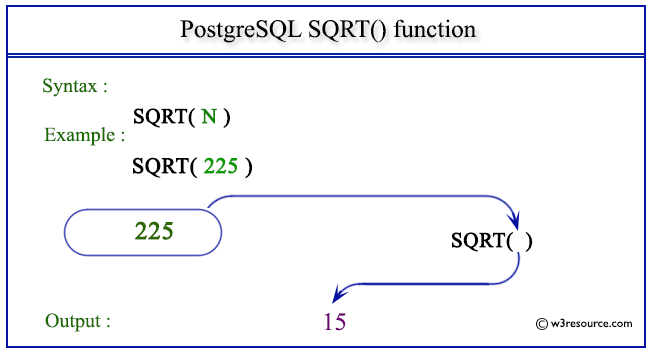# PostgreSQL SQRT() function

## SQRT() function

The PostgreSQL sqrt() function is used to return the square root of a given positive number.

Syntax:

```sqrt()
```

PostgreSQL Version: 9.3

Pictorial presentation of PostgreSQL SQRT() functionExample: PostgreSQL SQRT() function

Code:

``````SELECT SQRT(225) AS "Square Root";
```
```

Sample Output:

``` Square Root
-------------
15
(1 row)
```

Previous: SIN function
Next: TAN function

﻿